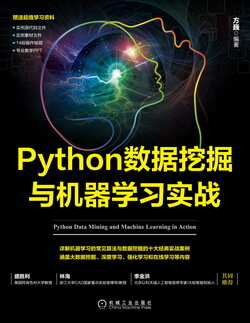# Python 数据挖掘与机器学习实战 (34)：Python 语言简介 2.5(Python 的元组)

Python 的元组与列表类似，不同之处在于元组的元素不能修改；元组使用小括号，列表使用方括号。元组的创建很简单，只需要在括号中添加元素，并使用逗号隔开即可。例如：

tup1 = ('a', 'b', 10, 20)tup2 = (1, 2, 3, 4, 5 )tup3 =("a", "b", "c", "d")tup1 = ()                                                       #创建空元组

tup1 = (50,)

tup1 = ('a', 'b', 10, 20)tup2 = (1, 2, 3, 4, 5 )print ("tup1: ", tup1)print ("tup2[1:4]: ", tup2[1:4])

tup1:  atup2[1:4]:  (2, 3, 4)

tup1 = (12, 34.56)tup2 = ('abc', 'xyz')# 以下修改元组元素操作是非法的# tup1 = 100# 创建一个新的元组tup3 = tup1 + tup2print (tup3)

(12, 34.56, 'abc', 'xyz')

tup1 = ('a', 'b', 10, 20)print (tup)del tupprint (" 删除后的结果: ")print (tup)

 ('a', 'b', 10, 20)删除后的结果:Traceback (most recent call last):  File "F:/program/2.5.py", line 26, in <module>    print (tup)NameError: name 'tup' is not defined

len 计算元素个数
+ 连接
* 复制
in 元素是否存在
for 迭代

tup1 = (1,2,3)tup2 = (4,5,6)print(len(tup1))print(tup1+tup2)print(tup1*3)print(3 in tup1)　for x in tup1:    print (x)

3(1, 2, 3, 4, 5, 6)(1, 2, 3, 1, 2, 3, 1, 2, 3)True123